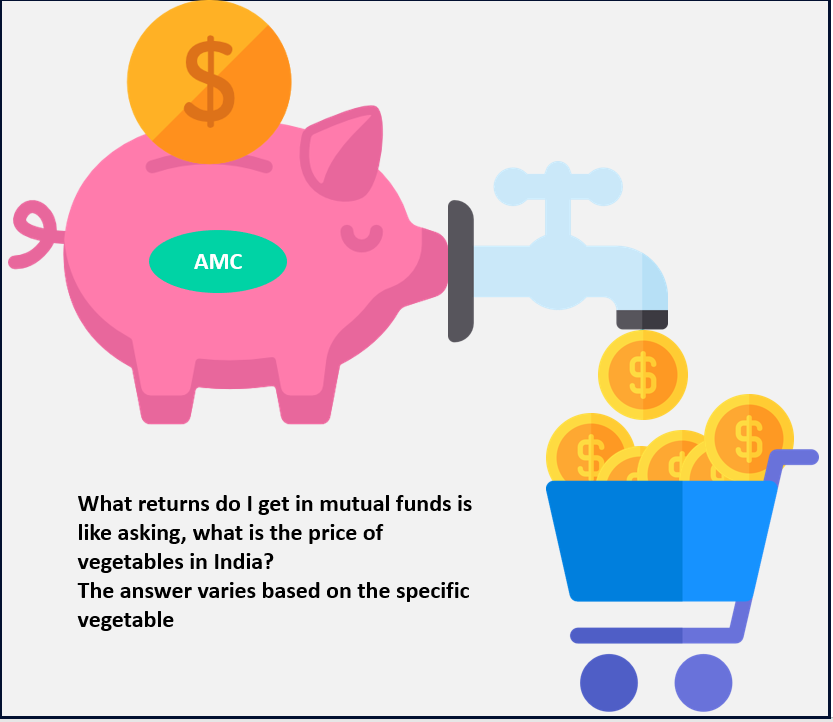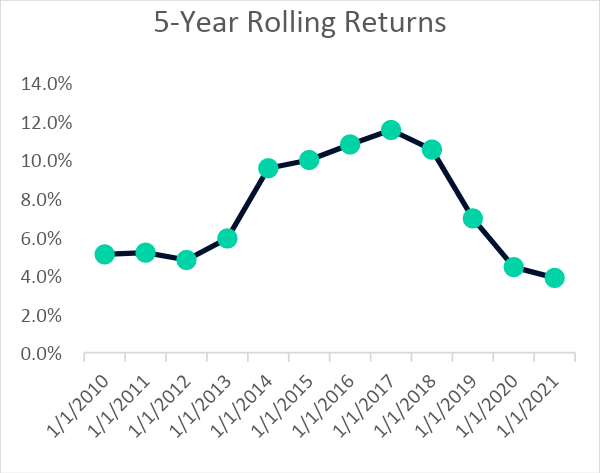# Demystifying Returns In Mutual Funds

Why do we invest in mutual funds? The fairly obvious answer would be to earn returns on our investment and to have enough corpus for our future goals. We need tangible numbers on our screens which give us a good night’s sleep that we have invested in the right fund.

However, there are multiple measures for the returns earned by the mutual fund, and we see multiple percentage numbers flashing on our screens. These measures are explained with examples in the following paragraphs –## 1. Absolute Returns

This represents the growth of your investment in absolute terms without considering the time period. For example, if you had invested Rs 10,000 in a mutual fund and it grows to Rs 15,000, the gain earned would be Rs 5000. Absolute returns would be Rs 5000/Rs 10,000 = 50%. Even if your investments grew to Rs 15,000 in 10 years the absolute returns would still be 50%.

## 2. Annualised Returns (also known as CAGR)

This measures the increment in the value of your investment on a yearly basis. The effect of compounding is included in this return (Compounding in simple terms is earning returns on the profits earned from your investment).

For example, if the initial investment is Rs 10,000 and the value of the investment after 5 years is Rs 15000, then the annualised returns would be 8.4% and if the time period was 3 years, the returns would be 14.5%. This measure takes the time period into consideration and gives a measure of y-o-y returns on your investment in the fund.

## 3. Annual Returns

This is computed by considering the return earned by the scheme from January 1st (first day of business) to December 31st (Last business working day). If the NAV of a fund was Rs 100 on January 1st and the NAV on December 31st was 120, the gain would be Rs 20 and the annual return would be 20%.

This is the most simplistic measure which is used for communication to the investor. Market conditions play a significant role in the returns earned by mutual funds. Hence, it is advisable to compare annual returns across time periods with respect to category average or the benchmark as declared by the fund.

## 4. Point to Point Returns

This measures the annualised returns between two points in a given time period. For example, you would want to look at the performance of a fund in the pre-Covid years i.e., 2017-2019, one would consider the point-to-point return to compute the same. The NAVs at the start and end dates are required to compute these returns.

## 5. Total Returns

Total return includes the returns earned from capital gains and dividends and is expressed as a percentage of the initial amount invested.

Consider that you had invested Rs 10,000 into a fund whose NAV was Rs 50.

Total Returns % = Capital Gains + Dividend earned / Initial Investment

Here, the total return earned would be 8%.

## 6. Trailing Returns

It is the annualised return of the period that ends on the date of calculation (or today or latest NAV). Trailing returns of 1, 3, 5 or 10 years (etc) can be calculated. For example, a 1-year trailing return from today (27th Feb 2020) would be calculated by taking the latest NAV and the NAV of the fund 1 year ago. This measure is used by most of the mutual funds and pages which analyse the past performance of the funds.

For example, if the NAV of a fund today is Rs 70 and the NAV of the fund 3 years ago was Rs 40, the trailing 3-year return would be 20.5%.

Returns = [Final NAV / Initial NAV] (1/Years) – 1

As an investor, this measure aids in screening the fund’s performance and analysing the consistency of the fund manager in providing the returns to their pool of investors. However, one should note that these returns could offer a biased perspective as they are based on relative market conditions – current vs past conditions.

Hence, an investor should consider 3,5 and 10 years to understand the consistency in earnings and the fund’s ability to sail over market tides. In bull markets, where there is high optimism in the market, the trailing returns are high, as the Final NAV would be soaring high, whereas, in the bear markets, these returns would be on the low.

## 7. Rolling Returns

These are annualised returns (CAGR) but are computed using overlapping periods. They give the measure of the growth of an n-year return over a period of m years.

For example, if you would like to invest in an equity mutual fund for 5 years, you would look at the data in 5-year blocks and compute the 5-year return over a 10-year period (say). As shown in the table below, we have considered a period from 2005-2020 to calculate the 5-year rolling returns (n=5, m=20).

Aligning with our objective, to calculate the return of 2010, we consider the NAV that was 5-years ago which is 2005. The exercise is performed for all the years to obtain the range of returns that the fund has given to the investors. One can also calculate the Rolling Return Average, by calculating the average of all the returns computed in the previous step = 7.4% (in this example).

The rolling returns give a perspective of the maximum and minimum ranges of returns that the fund has offered over a period of time, instead of only the point-point or annual returns, which could become biased measures based on the market conditions.These can be calculated on a daily/weekly/monthly basis till the latest NAV for a fixed period of time. It gives a more accurate picture of the fund’s performance in various market conditions, eliminating the bias that could be associated with calculating the return at a fixed point in time.

## 8. SIP Returns

All the above measures are suitable for lumpsum investing where one considers the returns between two points. However, in the case of SIPs, there is a systematic flow of amounts into the fund at different points in time. This return can be calculated using the Internal Rate of Return (IRR), which is a financial metric used to compute the return of a series of cash inflows and outflows.

## Conclusion

The measures for calculating returns have been highlighted above which are to be used in conjunction with the objective to obtain the accurate measurement of the performance. You could get started with your investment journey by analysing funds on the EduFund app or this website.